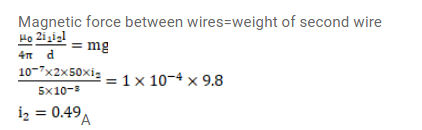# A long, straight wire is fixed horizontally and carries a current of

Question:

A long, straight wire is fixed horizontally and carries a current of $50.0 \mathrm{~A}$. A second wire having linear mass density $1.0 \times 10^{-4} \mathrm{~kg} / \mathrm{m}$ is placed parallel to and directly above this wire at a separation of $5.0 \mathrm{~mm}$. What current should this second wire carry such that the magnetic repulsion can balance its weight?

Solution: